# Reading and Interpreting a Line Graphs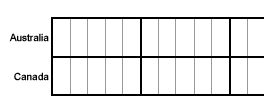# Understanding a Line Graph

Example 1: The table below shows daily temperatures for New York City, recorded for 6 days, in degrees Fahrenheit.

 Temperatures In NY City Day Temperature 1 43° F 2 53° F 3 50° F 4 57° F 5 59° F 6 67° F

The data from the table above has been represented in the graph below.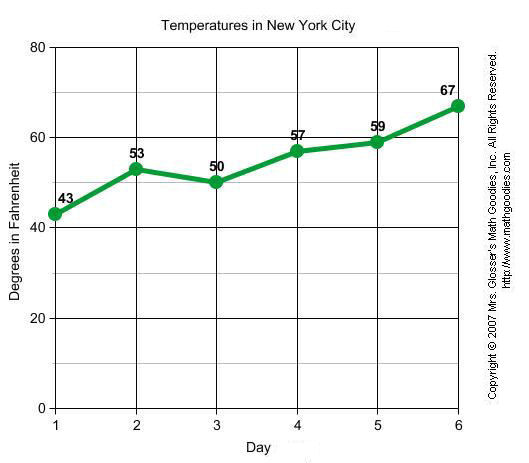Example 2: Sarah bought a new car in 2001 for \$24,000. The dollar value of her car changed each year as shown in the table below.

 Value of Sarah's Car Year Value 2001 \$24,000 2002 \$22,500 2003 \$19,700 2004 \$17,500 2005 \$14,500 2006 \$10,000 2007 \$ 5,800

The data from the table above has been represented in the graph below.Example 3: The table below shows Sam's weight in kilograms for 5 months.

 Sam's Weight Month Weight in kg January 49 February 54 March 61 April 69 May 73

The data from the table above has been represented in the graph below.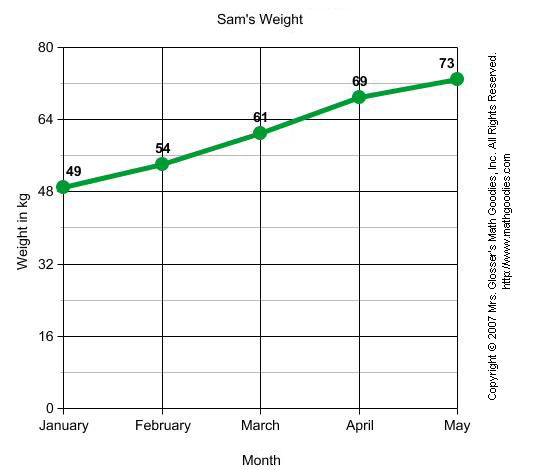In Example1, the temperature changed from day to day. In Example 2, the value of Sarah's car decreased from year to year. In Example 3, Sam's weight increased each month. Each of these graphs shows a change in data over time. line graph is useful for displaying data or information that changes continuously over time. Another name for a this type of graph is a line chart. The graph below will be used to help us define the parts of a line graph.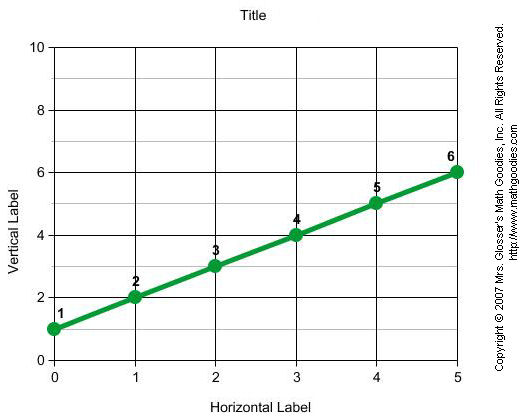Let's define the various parts of a line chart.

 title The title of the graph tells us what the graph is about. labels The horizontal label across the bottom and the vertical label along the side tells us what kinds of facts are listed. scales The horizontal scale across the bottom and the vertical scale along the side tell us how much or how many. points The points or dots on the graph show us the facts. lines The lines connecting the points give estimates of the values between the points.

Now that we are familiar with the parts of a line graph, we can answer some questions about each of the graphs from the examples above.QUESTIONANSWER 1. What is the title of this graph? Temperatures in New York City 2. What is the range of values on the horizontal scale?1 to 6 3. What is the range of values on the vertical scale?0 to 80 4. How many points are in the graph?6 5. What was the lowest temperature recorded?43° F 6. What was the highest temperature recorded?67° F 7. At what point did the temperature dip?Day 3: 50° FQUESTIONANSWER 1. What is the title of this graph? Value of Sarah's Car 2. What is the range of values on the horizontal scale?2001 to 2007 3. What is the range of values on the vertical scale?0 to 25,000 4. How many points are in the graph?7 5. What was the highest value recorded?\$24,000 6. What was the lowest value recorded?\$5,800 7. Did the value of the car increase or decrease over time?decreaseQUESTIONANSWER 1. What is the title of this graph? Sam's Weight 2. What is the range of values on the horizontal scale?January to May 3. What is the range of values on the vertical scale?0 to 80 4. How many points are in the graph?5 5. What was the highest value recorded?73 kg 6. What was the lowest value recorded?49 kg 7. Did Sam's weight increase or decrease over time?increase

Example 4: The graph below shows people in a store at various times of the day.QUESTIONANSWER 1. What is the graph about? People in a Store 2. What is the busiest time of day at the store?1 pm 3. At what time does business start to slow down?3 pm 4. How many people are in the store when it opens?2 5. About how many people are in the store at 2:30 pm?11 6. What was the greatest number of people in the store?22 7. What was the least number of people in the store?2

Example 5: The graph below shows the number of teens ages 13 through 19 in Smalltown that have cell phones.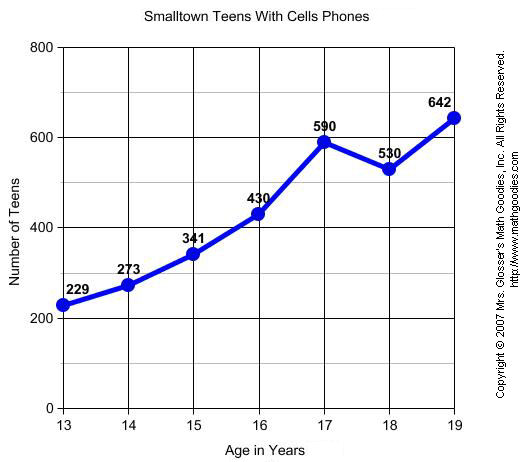QUESTIONANSWER 1. What is the graph about? Smalltown Teens With Cell Phones 2. At what age do teens have the greatest number cell phones?19 years 3. At what age do teens have the least number of cell phones?13 years 4. How many cell phones do 15 year-olds have?341 5. About how many cell phones do 16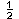year-olds have?500 6. What was the greatest number of cell phones at any age?642 7. What was the least number of cell phones at any age?229

Summary: A line graph is useful in displaying data or information that changes continuously over time. The points on the graph are connected by a line. Another name for a this type of graph is a line chart.

### Exercises:

Directions: Refer to the graph below to answer each question. For each exercise below, click once in the ANSWER BOX, type in your answer; and then click ENTER. Your answers should be given as a word or as a whole number. After you click ENTER, a message will appear in the RESULTS BOX to indicate whether your answer is correct or incorrect. To start over, click CLEAR.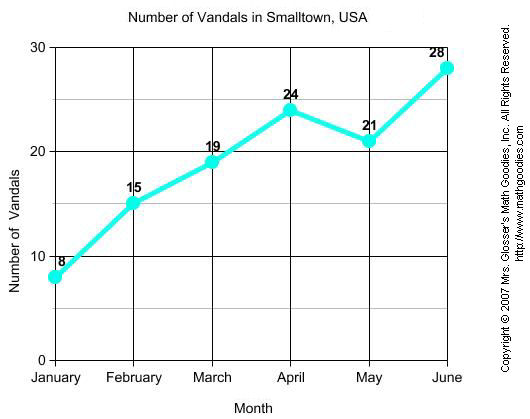1.In which month were there the most vandals? ANSWER BOX:    RESULTS BOX:
 2.In which month were there the least vandals?   ANSWER BOX:    RESULTS BOX:
 3.How many vandals were there in March? ANSWER BOX:    RESULTS BOX:
 4.In which month were there 24 vandals? ANSWER BOX:    RESULTS BOX:
 5.How many vandals were there in February? ANSWER BOX:    RESULTS BOX: